﻿ Antisymmetric wavefunction and Pauli exclusion principle

# Pauli exclusion principle ?

Summary

## Lithium = three 1s hydrogens ?

### [ Li of three 1s H wavefunctions gives lower energy than true energy. ]

(Fig.1)  ↓ If Li consists of 3 × H 1s wavefunctions ..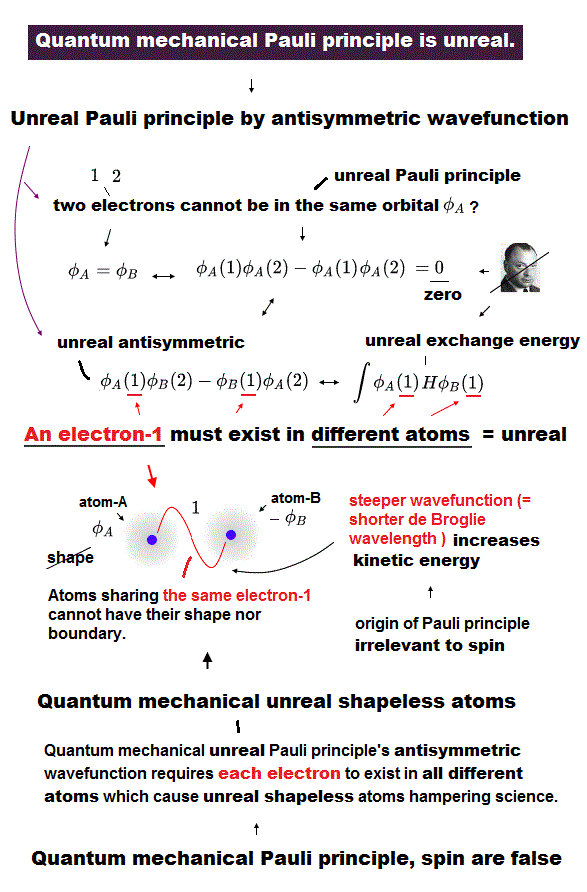Quantum mechanics claims that lithium (= Li ) cannot consist of three hydrogen 1s wavefunctions, instead, the 3rd electron of Li enter 2s orbital.

If lithium consists of three hydrogen 1s wavefunctions, it gives lower energy than true ground state energy, so unreal.

Li of 3 × H wavefunctions = -230 eV  ← lower than
True ground state energy of Li = - 203 eV

See this and use energy unit converter.

## Pauli exclusion principle by quantum mechanics.

### [ Antisymmetric wavefunction = Pauli exclusion principle ? ]

(Fig.2)  ↓ If two wavefunctions (φ=ψ) are the same, → zero ?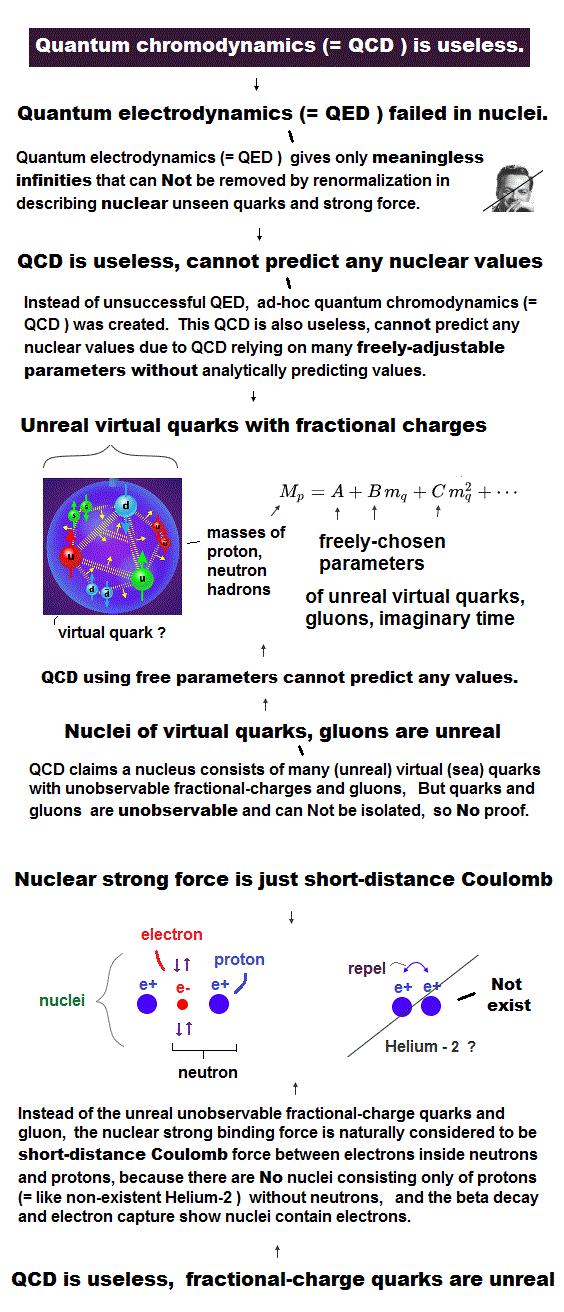Quantum mechanics introduced antisymmetric wavefunction as a reason for Pauli exclusion principle.

If two wavefunctions ( φ=ψ in Fig.2 ) are the same, the total wavefunction becomes zero, so any two wavefunctions must be different.  ← Pauli principle ?

Two 1s wavefunctions have different spins, up and down.
The 3rd Li has down (or up ) spin, so its wavefunction must be different from 1s.

But unreal electron spin disagrees with Pauli principle.

Quantum mechanics describes this Pauli principle = antisymmetric wavefunctin as determinant form.

## Quantum mechanics is wrong.

### [ If quantum mechanics is right,  Li = 3 × H is OK. ]

(Fig.3)  ↓ Wavefunction cannot be zero by "normalization"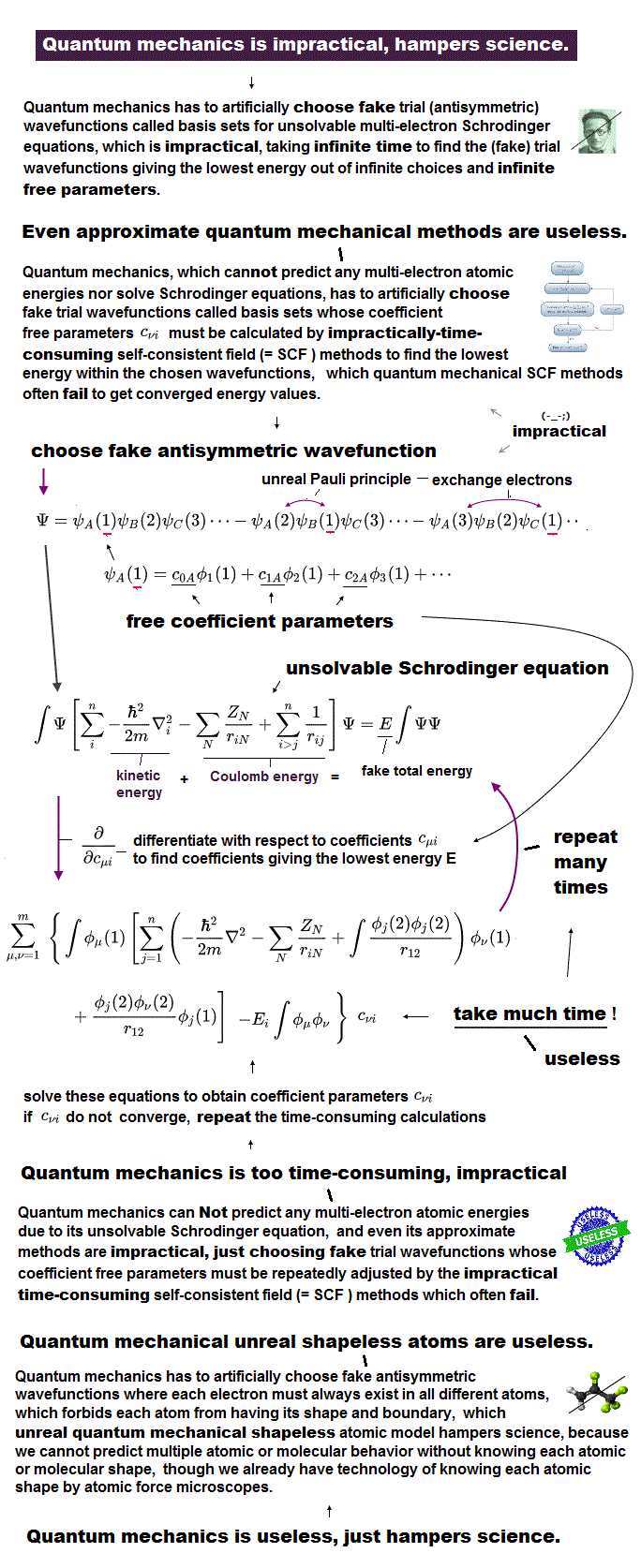Quantum mechanics claims, if two wavefunctions are the same, the total wavefunction becomes zero, this is Pauli principle.

But in fact, this quantum mechanical logic is wrong and inconsistent.

No matter how closer to zero the wavefunction becomes, this wavefunction is returned to "1 (= 0/0)" by normalization.

Even when the 3rd electron's wavefunction is closer to other 1s wavefunctions (= total wavefunction is closer to zero ), their total electron's probability must always be "1" by normalization ( this p.2 ).

In normarization, we devide the total wavefunction by its own wavefunction, so it cannot be zero, instead, its probability density always becomes 1.

So we can get the total energy E of lithium consisting of 3 × almost 1s H wavefunctions, which is unreal.  ← quantum mechanics is wrong.

By manipulating orbital exponent (= effective charge ) parameter, we can get any different hydrogen 1s wavefunction of slightly different atomic radius.

So if quantum mechanics is right, we can choose almost three hydrogen 1s wavefunctins as lithium solution, and it gives lower energy than true Li ground state energy, so wrong.

---------------------------

This quantum mechanical methods disagree with actual experiment.

In antisymmetric wavefunction (= triplet ? ), overlapped part (= electron repulsion is strong ) is cancelled, so triplet energies tend to be lower than singlet states, they claim.

Actual energy of 1s2s singlet (= 1S ) is lower than 1s2p triplet (= 3P ), but quantum mechanics gives lower 1s2p triplet than 1s2s singlet ( this last ).  ← opposite results !

The point is Schrödinger equation cannot have exact solution, so they just choose convenient wavefunction as fake solution which gives results closer to experimental values.

Artificial choice of these (anti)symmetric wavefunctions as fake solution is meaningless in a physical sense.

## Molecular bond by quantum mechanics.

### [ Electrons in quantum mechanics spread to "cloud" ? ]

(Fig.4)  ↓ An electron splits into infinitesimally smaller charges.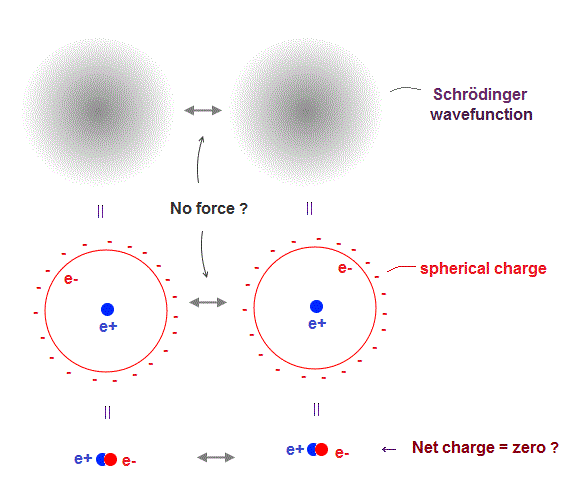In quantum mechanics, each electron splits into infinitesimally smaller charges and spread over all space, like "cloud".

Of course, actual electron does Not split into smaller charges.
But the quantum mechanical probability density is similar to electron cloud.

When an electron splits into smaller charges and is uniformly distributed over sphere, the potential is equal to the state when the point-particle electron is at the center of nucleus.

So even when an electron gets closer to another electron, it feels No repulsive force, because the total charge is always neutral zero (= electron + nucleus ).

This quantum mechanical electrons are Not reality.

## Molecular bond in quantum mechanics.

### [ Charges "penetrate" through electron cloud → attraction ? ]

(Fig.5)  ↓ small charges penetrate through spherical electron ?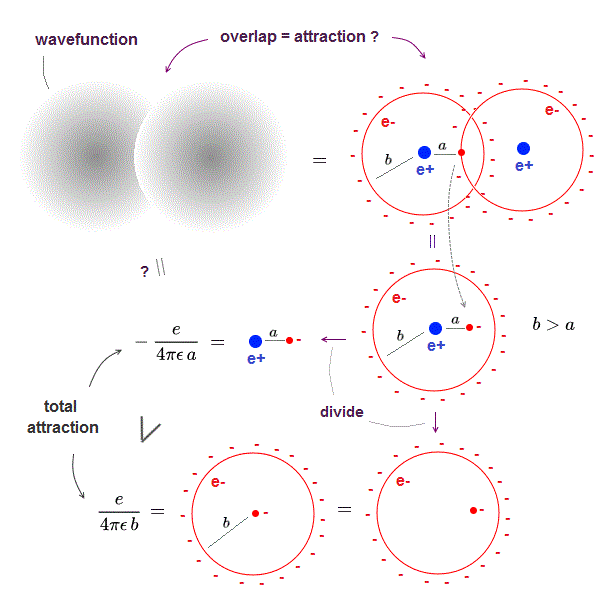When two electron clouds overlap, each small charges penetrate through another spherical electron cloud.

These small charges causes "attraction" in quantum mechanical molecule.

The distance (= a ) between positive nucleus and this charge is shorter than electron cloud radius (= b ).

So this small charge penetrating into spherical electron cloud feels more attraction from nucleus, which is stronger than repulsions from electron cloud.

As you see, intermolecular attraction (= molecular bond ) in quantum mechanics is caused by overlapped electron clouds (= a part of electron cloud penetrates another electron cloud ).

When these small charges go beyond another nucleus, repulsion between two nuclei become stronger than attraction between charge and nucleus, which limits the shortest internuclear distance.

This quantum mechanical molecule is Not reality.

## Hydrogen molecule (H2) in quantum mechanics.

### [ Overlapped wavefunction gives attraction ? ]

(Fig.6)  ↓ Antisymmetric wavefunction = Pauli repulsion ?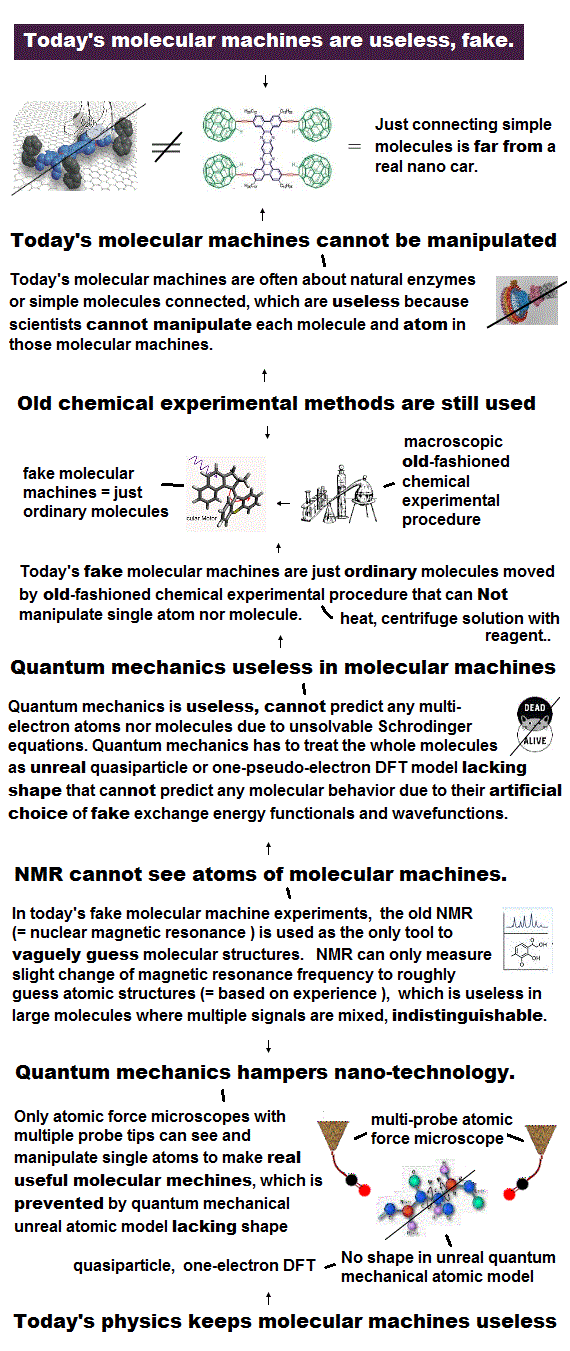Quantum mechanic uses two hydrogen atoms (= 1s wavefunction φ ) around different nuclei as hydrogen molecule (= H2, this p.3 ).

Overlapped part of two wavefunctions feels stronger attraction from another nucleus, which causes molecular bond energy in quantum mechanics.

When the entire wavefunction is antisymmetric, this overlapped part is cancelled ( minus - in Fig.6 ), which causes Pauli repulsion, they claim.

If this quantum mechanics is right, He2 dimer cannot exist.  But He2 dimer actually exists.

Schrödinger equation cannot have exact solution in any multi-electron atoms, so they just choose convenient wavefunction as fake solution.  ← meangingless.

## An electron is a "separated" charge !

### [ Quantum mechanics disagrees with actual molecules. ]

(Fig.7)  ↓ H-F ( F-Cl ) bond is stronger than H-Cl ( F-F ) = contradiction.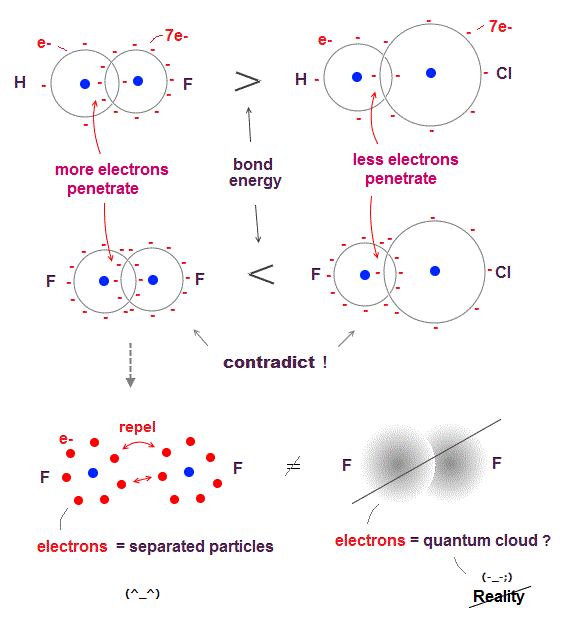According to quantum mechanics, attraction between molecules is caused by charges "penetrating" another electron cloud.

So when smaller atom contains more electrons (= denser charges ), it causes stronger molecular bonds in overlapped part, if quantum mechanics is right.

Fluorine (= F ) is more compact than chlorine (= Cl ), they have the same number (= 7 ) of valence electrons, so F electron charge is denser than Cl.

H-F ( or C-F ) bond energy is stronger than H-Cl ( or C-Cl ).  ← OK
But F-F ( or N-F ) bond energy is smaller than F-Cl ( or N-Cl ).  ← opposite !

The electron cloud of quantum mechanics cannot explain this contradiction, because electron cloud cannot distinguish each separated electron.

If we consider F contains seven "separated" electrons, F-F causes stronger repulsion (= smaller bond energy ) than F-Cl, which can explain quantum mechanical contradiction.

Each time quantum mechanical prediction is wrong, they just artificially choose different fake wavefunctions in different molecules out of infinite choices, which cannot predict anything.

## Pauli principle by quantum mechanics is wrong.

### [ If quantum mechanics is right, H3 molecule is possible !? ]

(Fig.8)  ↓ This is why quantum mechanics is wrong.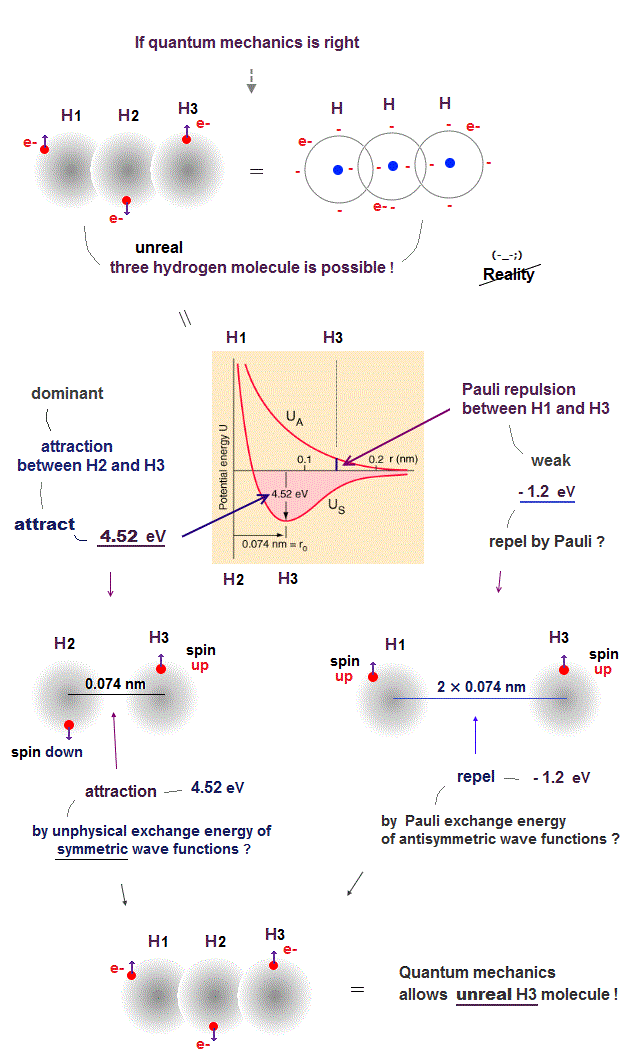We can prove quantum mechanics is wrong, because its unphysical "Pauli exchange energy" allows the existence of an unreal H3 molecule binding three hydrogen atoms.

According to unphysical quantum mechanical Pauli exclusion principle rule, when any two electrons' labeles are exchanged, the sign of the total wave function must be changed.

To satisfy this rule, the Schrödinger wave function must take the anti-symmetric form.

Each wave function consists of a spin component and spatial (= space ) component.

So if the spin component is antisymmetric (= one spin is up and the other spin is down ), the spatial (= space ) wave function must be symmetric.  → fake molecular attractive energy.

And when the spin component is symmetric (= both two spins are up and up,  or down and down ), the spacial wave function must be anti-symmetric.  → Pauli repulsive energy ?

Using this unphysical (spatial) symmetric wave functions of two hydrogen atoms, quantum mechanics allegedly obtains H2 molecular bond energy (= attractive energy ) close to 4.52 eV and its H2 bond length of 0.074 nm.

↑ In order to get this fake molecular bond attractive energy (= because any Schrödinger equations of multi-electron atoms or molecules cannot be solved ), quantum mechanics has to rely on unrealistic exchange energy by violating energy conservation law and artificially choosing fake wave functions.

Artificially choosing fake wave functions out of infinite choices and breaking inviolable physical principle of energy conservation law means quantum mechanics has No ability to predict any molecular bond energy in a realistic way, because quantum mechanical spreading electron clouds or wave functions cannot utilize ordinary Coulomb forces to bind two atoms ( this p.3-4 ).

This is why unphysical quantum mechanical "exchange energies" allegedly generating fake molecular attractive energies and Pauli repulstive energies are unreal, lacking real force or force carriers ( this p.11, this p.6 ).

There is No such thing as H3 molecule consisting of three hydrogen atoms.

So to prevent three hydrogen atoms from forming unreal H3 molecule, Pauli exclusion principle must prevent H2 and H3 atoms from binding, allowing only H1-H2 bonding.

Unfortunately, quantum mechanical Pauli exclusion principle caused by unphysical anti-symmetric wave functions is too weak to prevent this unreal H3 molecule formation.

H1 (= spin-up ) and H2 (= spin-down ) atoms have the opposite (= antisymmetric ) spins, hence H1 and H2 atoms can attract each other, and form H2 molecule due to the symmetric spatial wave functions.

In the same way, H2 (= spin-down ) and H3 (= spin-up ) can attract each other and form H2 molecular bond, hence the total wave function becomes unreal H3 molecule according to the quantum mechanical rule !

Because Pauli repulsive energy (= about -1.2 eV ) between H1 (= spin-up ) and H3 (= spin-up ) anti-symmetric spatial wave functions (= the distance between H1 and H3 atoms is twice longer than the distance between H2 and H3 atoms ) is far weaker than the attractive energy (= 4.56 eV ) between H2 (= spin-down ) and H3 (= spin-up ) symmetric wave functions, as shown in the upper figure ( this p.8-11,  this p.5 ).

This means the quantum mechanical unphysical rule of anti-symmetric wave functions causing fake molecular bond attractive energies and Pauli repulstive energies disagrees with the observed facts by allowing unreal H3 molecules, hence wrong.

We actually calculated using the quantum mechanical hydrogen molecular wavefunction and confimed quantum mechanics allows unreal H3, H4, H5 .. molecules combining more than two hydrogen atoms.

This is why Pauli repulsive energies between two molecules expressed as approximate Lennard-Jones potential (= these potential energy parameters must be atrificially determined by experiments, Not by quantum mechanical theory ) cannot be explained by quantum mechanics ( this p.3 left-middle,  this p.2 right-lower-half ).

## Coulomb repulsion between electron particles cannot be explained by quantum mechanical electron cloud.

### [ Quantum mechanics is unable to explain why F-F molecular bond energy is weaker than Cl-Cl bond. ]

(Fig.9)  ↓ Smaller fluorine (= F ) exerts stronger electrons' repulsions.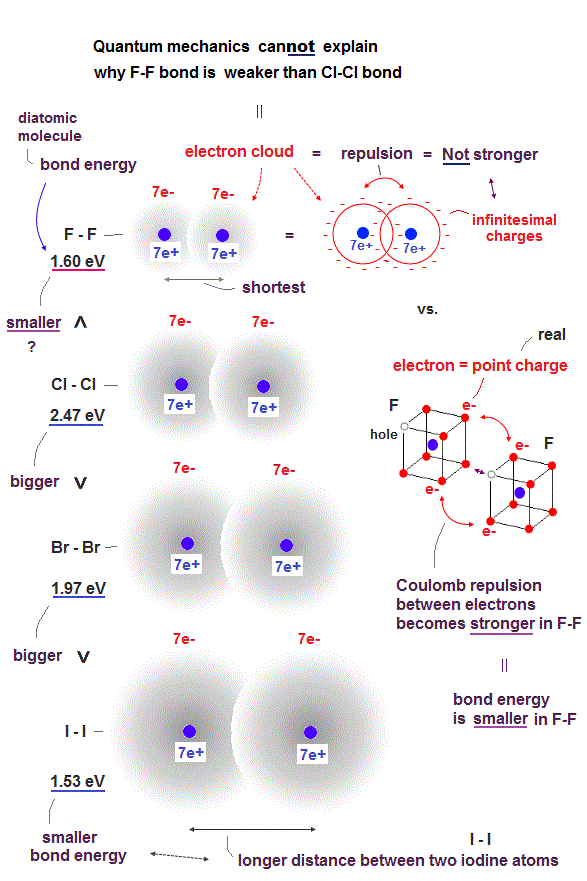Halogen atoms such as fluorine (= F ), chlorine (= Cl ), bromide (= Br ), iodine (= I ) are known to have the similar atomic orbitals and structure consisting of seven valence electrons.

Basically, it is known that smaller atoms tend to form shorter and stronger molecular bonds with bigger molecular bond energy (= H-F bond energy is bigger than H-Cl or H-Br molecular bond energies, because the F atomic size is smaller than Cl or Br atoms  → H-F molecular bond is shorter and stronger than H-Cl or H-Br ).

So molecular bond energies become smaller and weaker in bigger or longer diatomic molecules I-I and Br-Br than shorter Cl-Cl molecular bond.

But, strangely, the smallest halogen atom = fluorine (= F ) forms the weakest diatomic molecular bond (= F-F ) whose bond energy is far smaller than Cl-Cl molecular bond, though F-F bond length is shorter than Cl-Cl bond length (= F-F bond energy = 1.60 eV is smaller than Cl-Cl bond 2.43 eV or Br-Br bond 1.97 eV, as seen in this p.3 ).

It is natural to think this smallest and weakest F-F molecular bond is due to stronger Coulomb repulsions between electrons packed in the smallest space of the F atom.

The problem is quantum mechanical unphysical wavefunction or electron cloud cannot use real Coulomb force.

Quantum mechanical wavefunction or electron cloud is like a collection of infinite numbers of infinitesimal negative charges thinly spread all over the space into which a single electron particle is divided.

↑ Electron cloud is always a collection of inifinite number of inifinitesimal negative charges regardless of the atomic size or molecular bond length.

Hence, shorter F-F molecular bond (= which can be treated like a "contracted version" of Cl-Cl or Br-Br ) has to be stronger bond with bigger bond energy than longer Cl-Cl bond, if the quantum mechanical wavefunction or electron cloud is true.

So in order to explain paradoxical weaker F-F bond with smaller bond energy than longer Cl-Cl bond, each electron must be treated like a point-like charged particle instead of the spreading electron cloud consisting of infinite number of infinitesimal charges.

Because if each electron is a charged particle (instead of inifinitesimal charges ), shorter distance between such point-like electrons in shorter F-F molecular bond tends to exert stronger Coulomb repulsion, and as a result, shorter F-F molecule bond becomes weaker bound by smaller bond energy than longer Cl-Cl bond with weaker Coulomb repulsion between electrons which are more apart from each other.

Coulomb repulsion between two quantum mechanical wavefunctions or electron clouds, which are like uniformly charged spherical shells, cannot be stronger, no matter how closer and shorter the distance between two electrons clouds becomes.

No matter how close a outside electron charge gets to the surface of the uniformly charged spherical shell electron (= a collection of infinite number of inifinitesimal charges uniformly distributed over a spherical shell with radius R = quantum electron cloud ), the Coulomb repulsive energy between the outside electron and the surface of uniformly-charged electron cloud cannot exceed e2/R2.

If each electron is a point-like charged particle instead of the unphysical quantum mechanical electron cloud thinly spreading all over the space, as two electron particles approach each other, Coulomb repulsion between these two point-like electron particles can become very stronger.  ← This more realistic electron particle model can explain why shorter F-F molecular bond is weaker than longer Cl-Cl bond.

If the quantum mchanics is right, and each electron is like electron cloud = spherical shell uniformly charged with infinite number of infinitesimal negative charges, the outside electron cloud can easily penetrate the other electron cloud without being repelled by the infinitesimal electron's charges thinly distributed over the surface of the spherical shell.

As a result, it is impossible for the quantum mechanical wavefunction or electron cloud to explain why F-F bond is weaker than Cl-Cl bond based on stronger Coulomb repulsions between real electrons particles packed in smaller space of F-F molecule.

Only realistic atomic model treating each electron as a moving charged particle can explain this smaller F-F bond energy despite the shorter (= hence, usucally stronger bond ) F-F bond length.

## Quantum mechanics cannot explain the ordinary electric current.

### [ Electric current is explained only by "really-moving electron particles" Not by quantum mechanical static electron cloud. ]

(Fig.10)  ↓ Quantum electron cloud cannot explain electric current.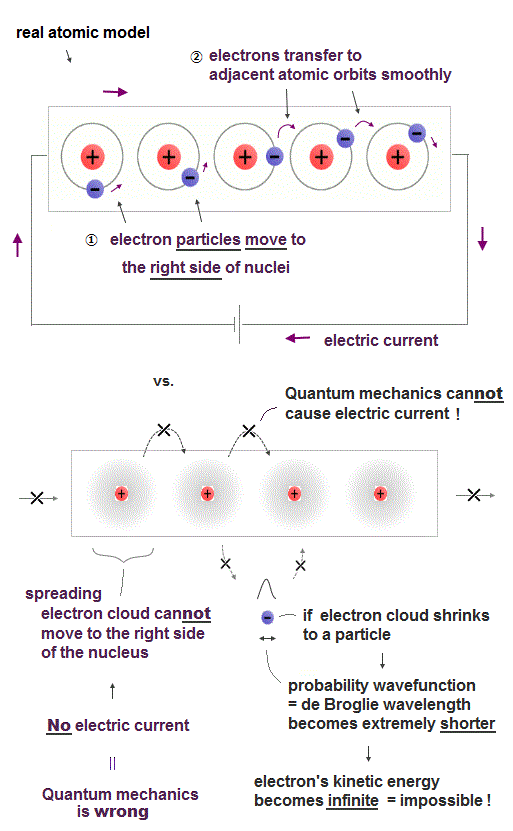Electric currents can be explained only by really-moving electron particles Not by unphysical quantum mechanical static electron cloud which must always spread all over space.

Because each electron must move from the left side to the right side of the nucleus, and smoothly hop into the adjacent atomic orbit without being excited (= energies required to excite atomic electrons are much larger than energies or voltage applied to the electric current ) when the electric current is flowing from the left to right.

But in the quantum mechanical wavefunction or electron cloud, each electron cannot move from left to right ( or right to left ) around the nucleus unlike a moving charged particle, hence, it's physically impossible for quantum mechanical electron cloud to cause real electric currents.

If the spreading quantum mechanical probability wavefunction or electron cloud tries to shrink to a moving particle's shape localized to the left or right side of the nucleus, its probability wavefunction or de Broglie wavelength becomes extremely shorter, and its electron's kinetic energy must become extremely higher.

It means an enormous amount of unnecessary energy is needed to cause electric current by quantum mechanical electron cloud model, which disagrees with how the actual electric current is generated.

This fact proves quantum mechanics and its unphysical electric cloud intrinsically cannot explain even the ordinary electric current, hence, quantum mechanics proved to be wrong.2021/8/16 updated. Feel free to link to this site.